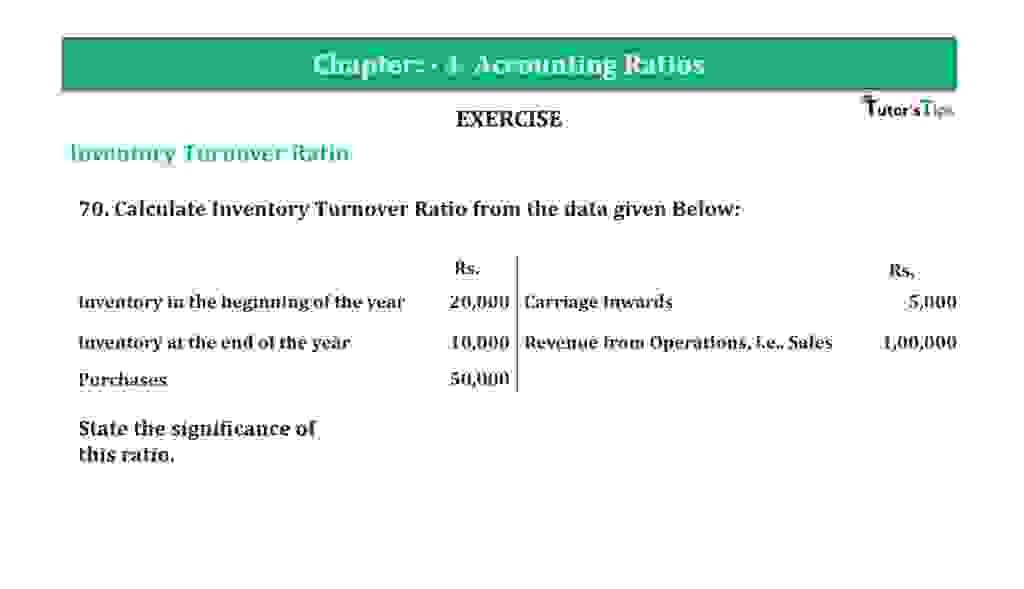# Question 70 Chapter 4 of +2-B – T.S. Grewal 12 ClassQuestion 70 Chapter 4 of +2-B

Interest Coverage Ratio

70. Calculate Inventory Turnover Ratio from the data given below:

 Rs. Rs. Inventory at the beginning of the year 20,000 Carriage Inwards 5,000 Inventory at the end of the year 10,000 Revenue from Operations, i.e., Sales 1,00,000 Purchases 50,000

State the significance of this ratio.

### The solution of Question 70 Chapter 4 of +2-B: –

 Cost of Goods of Goods Sold = Opening Stock +Purchases – Closing Stock = Rs. 20,000 + Rs. 50,000 + Rs. 5,000 − Rs.10,000 = Rs. 65,000

 Average Inventory = Opening Inventory + Closing Inventory 2

 Average Inventory = Rs. 20,000 + Rs. 10,000 2 = Rs. 15,000

 Inventory Turnover Ratio = Cost of Goods of Goods Sold Average Stock

 Inventory Turnover Ratio = Rs. 65,000 Rs. 15,000 = 4.33 Times

Balance Sheet: Meaning, Format & Examples

Thanks, Please Like and share with your friends

Comment if you have any question.

Also, Check out the solved question of previous Chapters: –

### T.S. Grewal’s Double Entry Book Keeping (Vol. II: Accounting for Companies)T.S. Grewal’s Analysis of Financial Statements